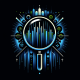flagshipdynamics
10/28/2018 - 4:09 AM

## Apply CRF - Image Segmentation

In this notebook I use Conditional Random Fields on the predicted masks. You can use it with your own submission and improve the score significantly by tinkering with this technique. Please refer the paper Conditional Random Fields as Recurrent Neural Networks for more information. Also, you may find this repo useful. Happy Kaggling :-)

``````import numpy as np
import pydensecrf.densecrf as dcrf
from skimage.io import imread, imsave
from pydensecrf.utils import unary_from_labels, create_pairwise_bilateral
from skimage.color import gray2rgb
from skimage.color import rgb2gray
import matplotlib.pyplot as plt
import pandas as pd
from tqdm import tqdm
%matplotlib inline

``````
``````def rle_decode(rle_mask):
'''
rle_mask: run-length as string formated (start length)
shape: (height,width) of array to return
Returns numpy array, 1 - mask, 0 - background

'''
starts, lengths = [np.asarray(x, dtype=int) for x in (s[0:][::2], s[1:][::2])]
starts -= 1
ends = starts + lengths
img = np.zeros(101*101, dtype=np.uint8)
for lo, hi in zip(starts, ends):
img[lo:hi] = 1
return img.reshape(101,101)``````
``````"""
reading and decoding the submission

"""
i = 0
j = 0
plt.figure(figsize=(30,15))
plt.subplots_adjust(bottom=0.2, top=0.8, hspace=0.2)  #adjust this to change vertical and horiz. spacings..
# Visualizing the predicted outputs
while True:
plt.subplot(1,6,j+1)
plt.title('ID: '+df.loc[i,'id'])
j = j + 1
if j>5:
break
i = i + 1``````
``````"""
Function which returns the labelled image after applying CRF

"""
#Original_image = Image which has to labelled
#Mask image = Which has been labelled by some technique..

# Converting annotated image to RGB if it is Gray scale

#     #Converting the annotations RGB color to single 32 bit integer

#     # Convert the 32bit integer color to 0,1, 2, ... labels.
colors, labels = np.unique(annotated_label, return_inverse=True)

n_labels = 2

#Setting up the CRF model
d = dcrf.DenseCRF2D(original_image.shape, original_image.shape, n_labels)

# get unary potentials (neg log probability)
U = unary_from_labels(labels, n_labels, gt_prob=0.7, zero_unsure=False)
d.setUnaryEnergy(U)

# This adds the color-independent term, features are the locations only.
d.addPairwiseGaussian(sxy=(3, 3), compat=3, kernel=dcrf.DIAG_KERNEL,
normalization=dcrf.NORMALIZE_SYMMETRIC)

#Run Inference for 10 steps
Q = d.inference(10)

# Find out the most probable class for each pixel.
MAP = np.argmax(Q, axis=0)

return MAP.reshape((original_image.shape,original_image.shape))``````
``test_path = '../input/tgs-salt-identification-challenge/test/images/'``
``````"""
visualizing the effect of applying CRF

"""
nImgs = 3
i = np.random.randint(1000)
j = 1
plt.figure(figsize=(15,15))
plt.subplots_adjust(wspace=0.2,hspace=0.1)  #adjust this to change vertical and horiz. spacings..
while True:
#Applying CRF on FCN-16 annotated image
plt.subplot(nImgs,4,4*j-3)
plt.imshow(orig_img)
plt.title('Original image')
plt.subplot(nImgs,4,4*j-2)
plt.subplot(nImgs,4,4*j-1)
plt.imshow(crf_output)
if j == nImgs:
break
else:
j = j + 1
i = i + 1``````
``````"""
used for converting the decoded image to rle mask

"""
def rle_encode(im):
'''
im: numpy array, 1 - mask, 0 - background
Returns run length as string formated
'''
pixels = im.flatten()
pixels = np.concatenate([, pixels, ])
runs = np.where(pixels[1:] != pixels[:-1]) + 1
runs[1::2] -= runs[::2]
return ' '.join(str(x) for x in runs)``````